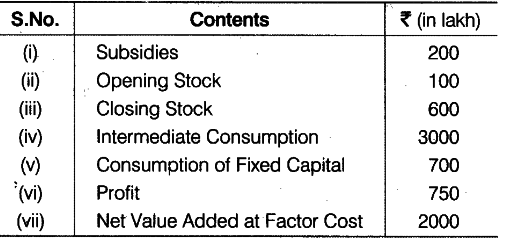# Calculate sales from the following data:

Calculate sales from the following data:Gross Value Added at Market Price (\$GV{{A}{MP}}\$)
= Value of Output - Intermediate Consumption
or
\$GV{{A}
{MP}}\$ =
Net Value Added at Factor Cost (\$NV{{A}{FC}}\$) - Subsidies + Consumption of Fixed Capital
\$GV{{A}
{MP}}\$ = 2000 - 200 + 700 = Rs. 2500 lakh
\$GV{{A}_{MP}}\$ = Sales + Change in Stock - Intermediate Consumption
2500= Sales + (600 - 100) - 3000
Sales = 2500 + 3000 - 500 = 5500 - 500 = 5000
Sales = Rs. 5000 lakh